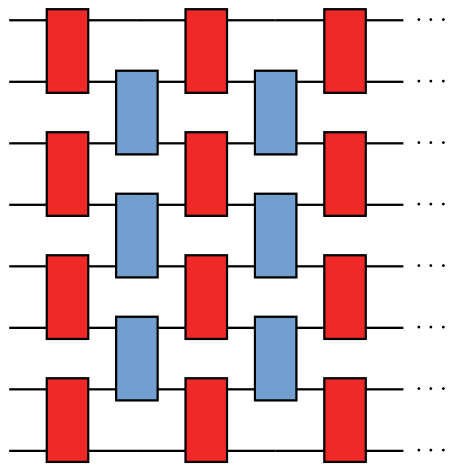# Nearly optimal lattice simulation by product formulas### Abstract

We consider simulating an $n$-qubit Hamiltonian with nearest-neighbor interactions evolving for time $t$ on a quantum computer. We show that this simulation has gate complexity $(nt)^{1+o(1)}$ using product formulas, a straightforward approach that has been demonstrated by several experimental groups. While it is reasonable to expect this complexity—in particular, this was claimed without rigorous justification by Jordan, Lee, and Preskill—we are not aware of a straightforward proof. Our approach is based on an analysis of the local error structure of product formulas, as introduced by Descombes and Thalhammer and further simplified here. We prove error bounds for canonical product formulas, which include well-known constructions such as the Lie-Trotter-Suzuki formulas. We also develop a local error representation for time-dependent Hamiltonian simulation, and we discuss generalizations to periodic boundary conditions, constant-range interactions, and higher dimensions. Combined with a previous lower bound, our result implies that product formulas can simulate lattice Hamiltonians with nearly optimal gate complexity.

Type
Publication
Phys. Rev. Lett. 123, 050503 (2019)##### Yuan Su
###### Research Scientist

I work on quantum algorithms for simulating Hamiltonian dynamics. I am particularly interested in the design, analysis, implementation, and application of quantum simulation.